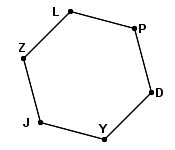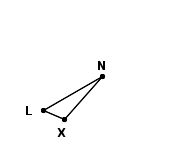edHelper subscribers - Create a new printable

Number of Keys
 Select the number of different printables: 1 key 2 keys 3 keys 4 keys 5 keys

Number of Pages (for each key)
 Select the number of pages: 1 page 2 pages 3 pages 4 pages

Include an answer key (answer keys will be at the end of the printable)

Sample edHelper.com - Math Review Worksheet

 Name _____________________________Date ___________________
Mixed Review
Divide.
1.
302,181

2.
90314

3.
605,459

4.
40466

5.
40608

6.
701,850

Find the value of n.
7.Regular polygon JZLPDY:YJ
=  33 in
Perimeter = n
8.Polygon NXL:NX
=  48 mmXL
=  19 mmLN
=  n
Perimeter = 124 mm

Circle the mixed number which is equal to the improper fraction.
9.
 259

2
 79
2
 29
25
 49
3
 79
10.
 275

6
 25
5
 25
4
 12
1
 25

 Key #2
 11 Cameron picked two and a half bushels of corn yesterday and four bushels today. How many bushels of corn did Cameron pick in all?
 12 Austin jumped four and a half feet for the long jump on Field Day. Tyler jumped six and one-fourth feet. How much further did Tyler jump than Austin?

Write each as a decimal.
13.
7
 2521000
 14 nine and one hundred thirty-six thousandths
15.
4
 710
 16 five and nine hundred sixty thousandths
17.
70
 410
18.
8
 10100
 19 two and nine hundredths
 20 six and sixty-three hundredths

Write an equation for each problem. Then solve the equation.
 21 84 divided by a number is 12.
 22 A number divided by 10 is 2.
 23 11 plus a number is 33.
 24 A number minus 69 is 27.
 25 A number plus 17 is 55.
 26 The difference between 47 and a number is 4.

Complete.
 27 4.3  -  4.118
 28 90.08  -  23.7
 29 4.05  +  82.027
 30 8.09  +  7.023
 31 31.3  -  9.4
 32 69.041  +  1.4

edHelper subscribers - Create a new printable

Number of Keys
 Select the number of different printables: 1 key 2 keys 3 keys 4 keys 5 keys

Number of Pages (for each key)
 Select the number of pages: 1 page 2 pages 3 pages 4 pages